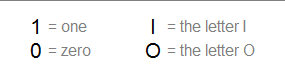# How to Convert to Base-n with the Remainder Method

 Base-n Conversion Calculator Base-10 Number = Convert to Base--2345678910 1112131415161718192021222324252627282930313233343536For any integer n greater than 1, there is a simple procedure to convert a base-10 (decimal) number to its base-n representation using nothing more than elementary division with remainders. This is faster than the technique of dividing by successively smaller powers of n.

If n is greater than 10, you will need to introduce more symbols to augment the standard set of ten digits. For example, in base-16 (hexadecimal), you use the digits 0-9 plus the letter-digits A-F to represent 10 through 15. Numeral systems with bases greater than 10 have shorter strings of digits, while those with bases less than 10 have longer strings. For instance, the decimal number 1777 is 6F1 in base-16 and 11011110001 in base-2.

The remainder method for base conversion is explained below so that you can convert to any number system by hand. For n = 2 through 36, you can use the calculator on the left. The letter-digits A-Z represent the numbers 10 through 35.

### Converting to Base-n With Remainders

This technique outputs the digits of the number in reverse order, so when the algorithm finishes, you simply read the digits from end to beginning to obtain the number. As an example, let's convert the decimal number 134,907 to base-20. We will need the digits 0-9 plus the letter-digits A-J to represent 10 through 19.

First, divide 134,907 by 20. This gives you a quotient of 6,745 and a remainder of 7. The number 7 will be the last digit of the final answer.

Now take 6,745 as the new dividend and divide it by 20. This gives you 337 with a remainder of 5.

Continuing with the process, take 337 as the new dividend and divide by 20 to obtain a quotient of 16 and a remainder of 17 = H.

Finally, 16 divided by 20 equals 0 with a remainder of 16 = G. Once you get a quotient of 0 you can stop.

Reading the list of remainders from top to bottom gives us the base-20 form of 134,907 as GH57.